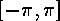<b>double atan2(double y, double x)</b>

Document: The C Standard Librarydouble exp(double x)Mathematical Functions: math.hdouble atan(double x)

## double atan2(double y, double x)

The return value is the inverse tangent or arctangent of y/x. The return value will be expressed in radians and lie in the interval.

The advantage of atan2() over atan() is that, because it is given separate access to the two original (rectangular) co-ordinates, it is able to distinguish beween angles in the left and right half planes (which is not possible if only y/x is passed in, as is the case with atan()).

McMullin@eeng.dcu.ie
Fri Mar 29 14:35:38 GMT 1996